# W-function of Lambert and its applications

## Introduction

Mathematical analysis knows many beautiful functions with unusual properties. Among them are integral sine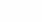and logarithm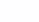also note the gamma functionor the very famous Riemann zeta function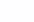. But today I invite the reader to look at the W-Lambert function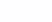## What is the Lambert W-function?

In order to understand what the Lambert W-function is, it is enough to look at the following equality, which, by analogy with the basic trigometric identity, I propose to call the “basic Lambert identity”: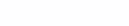In other words, the Lambert function is the inverse of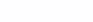. However, after the first studies, it becomes clear that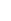not injective, but exactly the same meaning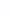is achieved with two different arguments if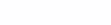. Therefore, the above definition needs some explanation.

By examining the derivative of the function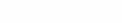we understand that the function increases by[-1; +\infty)” alt=”[-1; +\infty)” src=”https://habrastorage.org/getpro/habr/upload_files/788/259/543/788259543709a1e97476adfe3066d2a7.svg” width=”82″ height=”22″/>и убывает на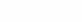. Thus, let’s construct the inverse function to the given one on the corresponding intervals of monotonicity.Plots of the Lambert W-function (black) and y = xe^x (grey)

The branch for which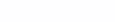is called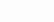another –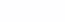.

## Formulation of the problem

Task. Learn to find the real roots of an equation of the following form: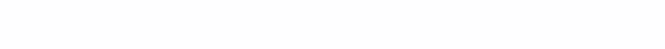As you probably already guessed, we will use the Lambert W-function for the solution. So, first we raise both the left and right sides to a power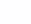(this transformation is not equivalent for even integers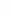and for odd ones, it will be necessary to expand the set of values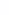to all real numbers, so we solve the problem for the above restrictions).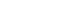Now, in order to use the basic Lambertian identity, we need to obtain an expression with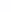the same as in the exponent. To do this, we divide both the left and right sides by.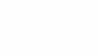And now we can use the basic Lambertian identity: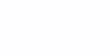From where we get the final formula for.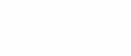## Calculation of the Lambert W-function

Note that when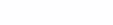the Lambert function gives two real values: one on each of the branchesandrespectively. In this case, the original equation will have 2 roots.

Calculating W0. We will use the method binary search by answer. We can do this becauseincreases by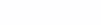.

The left bound of binary search is clear and equal to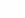. Now the question arises how to choose the right border. The first idea that comes to mind is to put it equal tobecause the inequality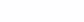and equality is achieved only at zero.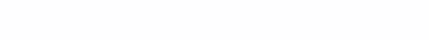However, for large enoughthis may not be the best option. So let’s look at another one: we choose the right border equal to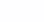.Total: at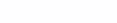choose the right borderand when.

Asymptotics: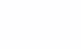prec – initially chosen precision (for example, 10-12)

Calculating W-one. Here we will use the following infinite expression for: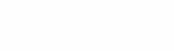The deeper we go down, the higher the accuracy of the calculations.

## Implementation in Python

from math import *

def LambertW0(x):
left = -1
right = x if x <= e else log(x)
prec = 10**-12 # точность
# бинарный поиск
while right - left > prec:
mid = (right + left) / 2
if mid * exp(mid) > x:
right = mid
else:
left = mid
return right

def LambertW_1(x, t): # t - показатель точности
if t == 100:
return log(-x)
else:
return log((-x)/(-LambertW_1(x, t + 1)))

def sol(p, q):
s = q**(1/p) / p
if s < -exp(-1):
return "No real solutions"
ans = "Solutions: " + str(p * LambertW0(s)) + " "
if -exp(-1) < s and s < 0:
ans += str(p * LambertW_1(s, 0))
return ans

p = float(input())
q = float(input())
print(sol(p, q))

## Examination

Equation 1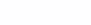Equation 2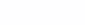Equation 3